Next: Expansion of orbital evolution Up: Derivation of Lagrange planetary Previous: Lagrange planetary equations

# Alternative forms of Lagrange planetary equations

It can be seen, from Equations (G.115)-(G.120), that in the limit of small eccentricity,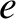, and small inclination,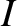, certain terms on the right-hand sides of the Lagrange planetary equations become singular. This problem can be alleviated by defining the alternative orbital elements,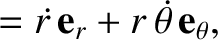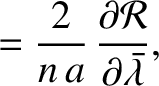(G.121)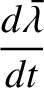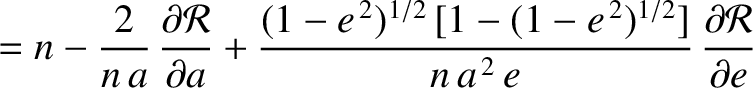(G.122)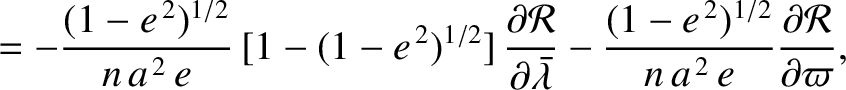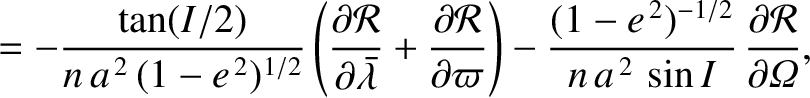(G.123) and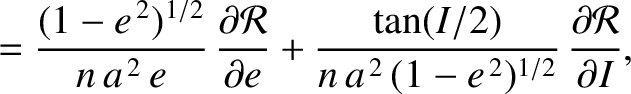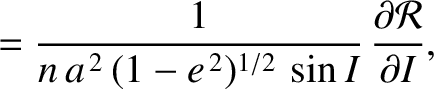(G.124)

If we write the Lagrange planetary equations in terms of these new elements then we obtain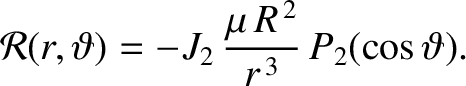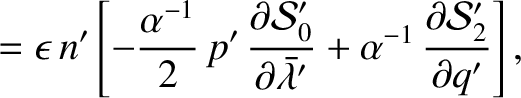(G.125)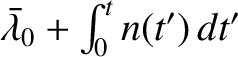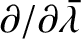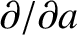(G.126)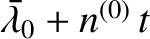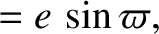(G.127)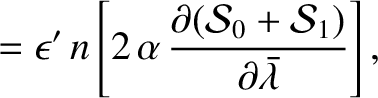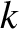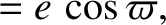(G.128)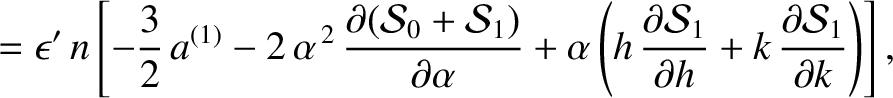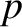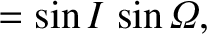(G.129) and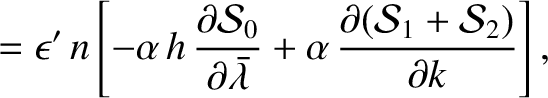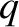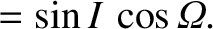(G.130)

Note that the new equations now contain no singular terms in the limit.

It is sometimes convenient to write the Lagrange planetary equations in terms of the mean anomaly,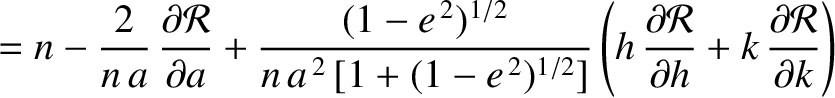, and the argument of the perigee,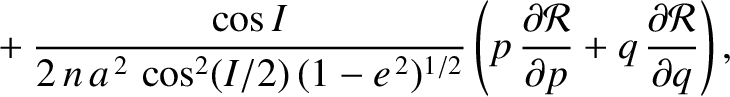, rather than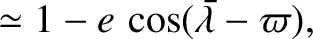and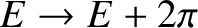. Making the appropriate substitutions, the equations take the form (Brouwer and Clemence 1961)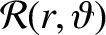(G.131)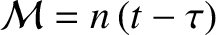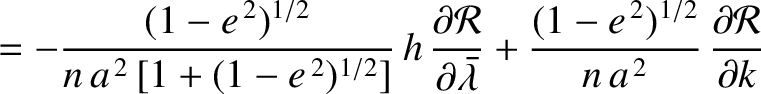(G.132)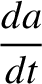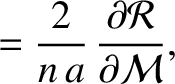(G.133)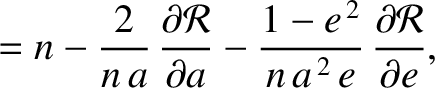(G.134)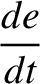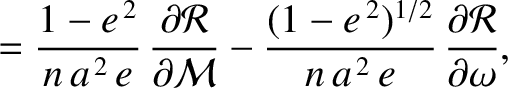(G.135) and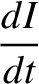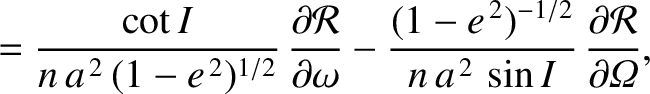(G.136)Next: Expansion of orbital evolution Up: Derivation of Lagrange planetary Previous: Lagrange planetary equations
Richard Fitzpatrick 2016-03-31